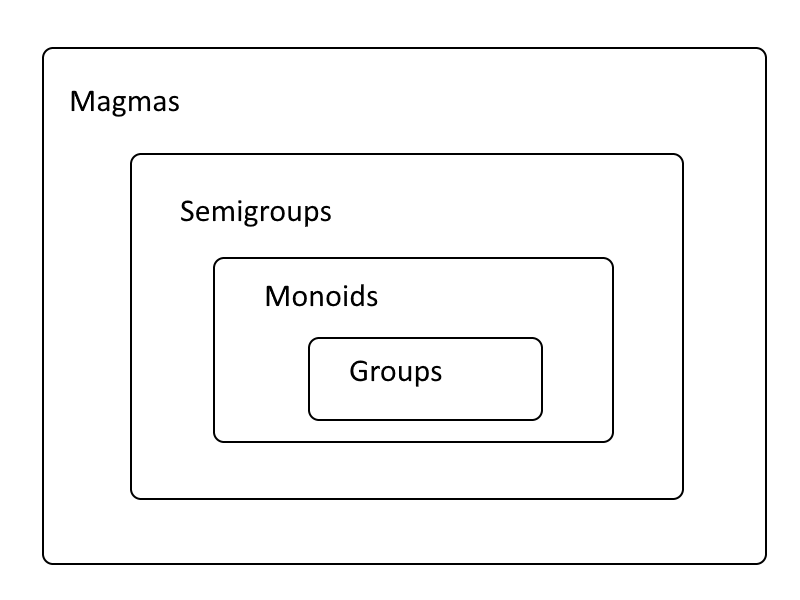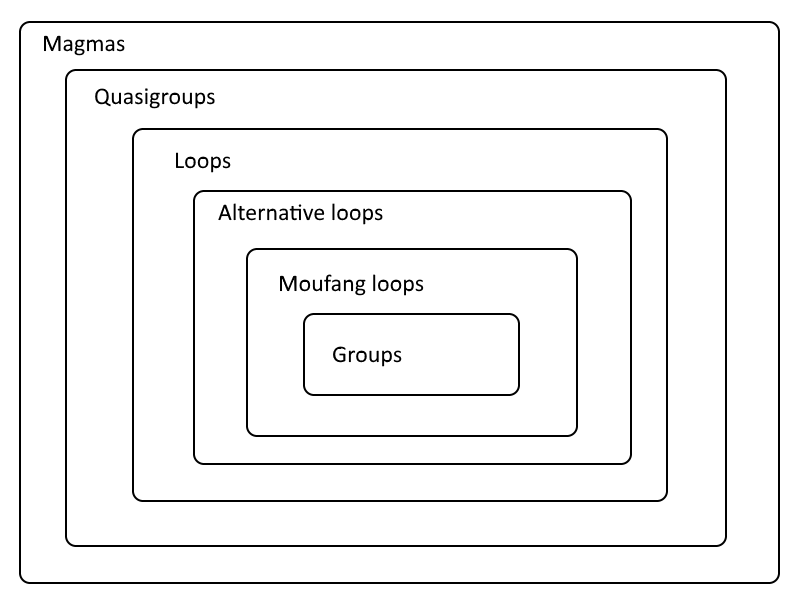# Weakening the requirements of a group

A group is a set with a binary operation that

1. closed
2. associative,
3. has an identity, and
4. has inverses.

This post will look at each of these properties and what happens if you modify or remove it.

## Magmas

Closed means that applying the binary operation to any two elements of the set yields another elements of the set. (I saw a book one time that had at the top of some chapter a quote something like “The product of two real numbers is a real number; we should be surprised if it were a head of cabbage.”) For example, the positive integers are closed under multiplication but not under division.

A set with a closed binary operation is called a magma.

## Semigroups

Associative means that you can parenthesize an expression any way you want and always get the same result. It may be practically important how you parenthesize an expression. For example, matrix multiplication can far fewer operations if you carry out the multiplications in the right order. In programming, left folds and right folds (such as `foldl` and `foldr` in Haskell) may have very different efficiency, but they’re supposed to return the same result if you’re folding an associative operation.

A magma with an associative operation is called a semigroup.

Differential equation theory is concerned with analytic semigroups. If you advance the solution of an evolution equation by a positive time increment t and then by an increment s, you end up at the same place as if you’d advanced the solution by ts.

Notice that the time increment is required to be positive. That’s because the initial conditions for an evolution equation might reside in a different function space than the solutions at any future time. That’s the case, for example, with the heat equation, where initial conditions can be rough but the solution smooths out immediately as you move forward in time.

## Monoids

If you add the requirement that a semigroup has an identity, you get a monoid. Partial differential equation theory is more concerned with analytic semigroups than analytic monoids for the reason explored above: you might not have an identity.

Positive integers with multiplication form a monoid but not a group because they have an identity, 1, but positive integers other than 1 do not have a multiplicative inverse that is an integer.

A monoid in which every element has an inverse is a group.## Quasigroups

So far we’ve built up a progression of stronger and stronger requirements leading up to groups, starting with requiring that our operation is associative. What if we don’t have associativity, but we do have other properties?

Non-associative operations are less common. Perhaps the best known operation that is not associative is octonion multiplication.

There are four division algebras over the reals: the reals themselves, complex numbers, quaternions, and octonions. Real and complex numbers are both fields. Quaternions are not a field because multiplication is not commutative. Octonions are even further from being a field because multiplication is not even associative.

But division is possible for octonions, except for 0, and so non-zero octonions form a quasigroup, a magma with division.

## Loops

A quasigroup that has an identity element is called a loop. Note that for quasigroups we said “division is possible,” not that elements have inverses. If elements do have inverses, then you can divide by an element by multiplying by its inverse. But you can define division by the ability to solve equations. We say you can divide b by a if axb and yab have unique solutions.

A loop with associativity is a group.

## Alternative loops

Loops are not required to have an associative property, but they may satisfy a weak form of associativity.

An alternative loop satisfies x(xy) = (xx)y and x(yy) = (xy)y. Non-zero octonions form an alternative loop with respect to multiplication.

## Moufang loops

A Moufang loop is a loop that satisfies these requirements:

1. z(x(zy)) = ((zx)z)y
2. x(z(yz)) = ((xz)y)z
3. (zx)(yz) = (z(xy))z
4. (zx)(yz) = z((xy)z)

These conditions imply the alternative loop conditions, so Moufang loops are alternative loops.

Moufang loop are still not required to be associative, but they’re getting closer. And as you might have anticipated, non-zero octonions with respect to multiplication form a Moufang loop.